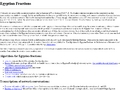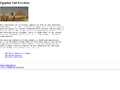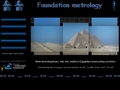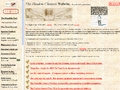EGby.net Egypt# Egypt, History By Time Period Mathematics

EgyptAncient Egyptian Mathematics - Some comments on Egyptian mathematics and the Rhind papyrus.Egyptian Fractions - Egyptian mathematical fractions, including algorithms, transcriptions of network conversations, and links to other Egyptian mathematical resources.Egyptian Fractions - Article from MathWorld describing unit fraction methods.Egyptian Math - Brief information on the decimal system using seven different symbols.Egyptian Mathematics - An overview of mathematics in Ancient Egypt. Includes descriptions of the numerals used and of some major papyri.Egyptian Unit Fractions - Articles concerning the mathematics of Ancient Egypt, from "MathPages."Foundation Metrology - New investigations into the earliest Egyptian measuring systems.The Pythagorean Theorem - Theory that the Pythagorean theorem was developed in EgyptThe Shadowchasers - Information and theories on numbers, mathematics and scientific tools.

Egypt